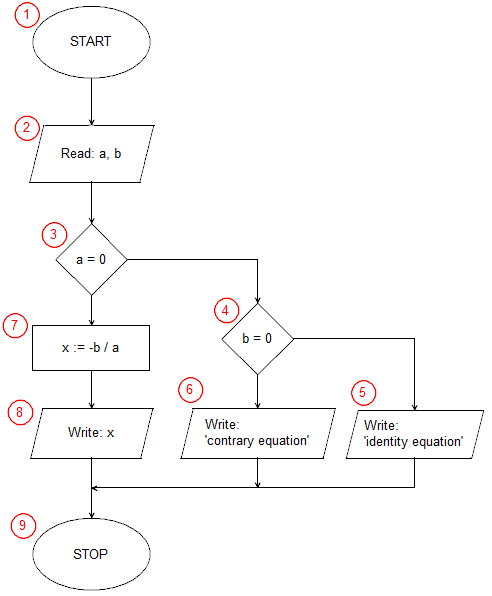# Implementation of a Linear equation solving algorithm in JavaScriptIn the 1st line of the program, we inform the browser that our document was written in HTML (more precisely, it is an HTML 5 header). Line 2 contains the <html> tag that begins the HTML code. The 3rd line starts the <body> section of our document, containing the elements that are to appear on the page. In our example, the <body> section contains only a script written in JavaScript that implements the algorithm for solving a linear equation. The <script> tag from line 4 informs about the start of the script - we can treat it as the starting block (1) of our algorithm. The content of the script is contained in lines 5 to 13.

In line 5, we declare the variable a with the var keyword, while the assignment operator ("=") initializes this variable with the value entered by the user using the prompt() statement. prompt - JavaScript standard function - displays a text input pop-up on the screen. The first argument of the function specifies the text displayed as a prompt, the second is the default value entered in the edit field. In our case, we're displaying "a:" as a prompt, and we're not prompting the user with any default text (the second argument is the empty text ""). As a result, the prompt() function returns the text entered by the user into the edit field and then confirmed with the OK button.

In line 6, we initialize the variable b in a similar way. Lines 5 and 6 correspond to the input/output block (2) of the discussed algorithm.

The conditional statement on line 7 is the selection block (3) of our algorithm. The instruction checks whether the coefficient a is equal to 0. When the condition is met, the instruction after the keyword then, written in lines 8 and 9 (in the algorithm, the selection block (4) and input-output blocks (5) and (6)) is executed. When the condition is not met, the statement after the else keyword is executed, in our case it is a complex statement, enclosed in braces between lines 10 and 13. First, in line 11, we calculate the solution to the equation x = -b/a (operation block (7)), and then in line 12 we write the result (input-output block (8)).

The </script> tag from line 14 terminates the program (algorithm end block (9)).

Then, in lines 15 and 16, we close the </body> and </html> sections.